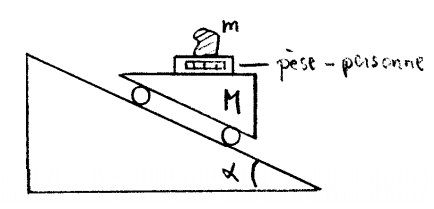# Inertia and moving plane problem

• gillouche

#### gillouche

Gold Member
Hi,

I have some troubles to understand the next step for the solution. If you need more informations, please let me know.

1. Homework Statement

A moving vehicle of mass M is moving down an inclined plane of angle alpha with respect to the horizontal plane. What is the force indicated on the scale ?It is written pèse-personne between M and m. That's a scale (to weight people)

## Homework Equations

[/B]
Acceleration of M on the inclined plane : a = g*sin(alpha) * (M/m + 1)

## The Attempt at a Solution

[/B]
I started to try to find the acceleration of M on the inclined plane. I have the following forces acting on my moving vehicle : gravity (down), normal force (perpendicular to the inclined plane) and the weight of the mass m (it is not indicated but I assume that the scale doesn't have a mass).

I separated the gravity components in parallel and perpendicular component and I get :

Fg parallel inclined plane = (M+m)g * sin(alpha)
The Fg perpendicular cancels out with normal force. I don't think I need it but that's the same of Fg parllel but with cosine.

Then I have ma = (M+m)g * sin(alpha) which gives me a = g*sin(alpha) * (M/m + 1)

(I can give the steps if needed).

Now I am stuck. How can I find the normal force for m ?

For m, I have the weight (down), the normal force (up), acceleration (right) and inertia (left). Those orientations are with respect to the moving vehicle and not the inclined plane. Am I supposed to break all the components in their parallel and perpendicular (to the inclined plane) components ?

I am confused with this inclined and not inclined plane of the moving vehicle. The exercises we did before was an elevator (for the weight) and a moving vehicle on the horizontal axis (to introduce inertia).

Thanks.

Perhaps take a fresh look at this.

Do you think horizontal motion will affect the reading on the scales?

No, only the gravitational force acting on m + the vertical acceleration that m has (other than g).

So I have my acceleration to the right parallel to the inclined plane, I should find the total acceleration perpendicular to the horizontal plane for m.

If horizontal motion is not relevant, then how can you simplify the problem?

Hint: Acceleration is a vector.

I know that my acceleration vector for the moving vehicle is parallel to the inclined plane. I can use the angle alpha to break it into x and y components. For the y component (that's the one I need for the vertical acceleration), I get g * sin2(alpha) * (M/m + 1)

If I look at the forces acting on my mass m. Is that correct to say that I have the following :

ma = mg + m * (perpendicular acceleration component of a) - normal force for m

So I should replace the "a" by the acceleration of M on the inclined plane and "perpendicular acceleration component of a" then simplify ?

I did it and I get mg(1 - sin(alpha) * (M/m + 1) + sin2 * (M/m + 1)))

The answer I get seems weird.

I can't understand why you think M is relevant. If you are standing on scales in your house, is the mass of your house relevant?

I can't understand why you think M is relevant. If you are standing on scales in your house, is the mass of your house relevant?
No but the acceleration of the whole system depends on M too. Am I wrong ? Maybe my calculation for the acceleration of the whole system is wrong.

No but the acceleration of the whole system depends on M too. Am I wrong ? Maybe my calculation for the acceleration of the whole system is wrong.
The acceleration of the whole system depends neither on M nor m. Only on g and the angle of inclination.

And the force m exerts on the scales cannot depend on the mass underneath the scales.